Product (C) of the reaction is:-

(a)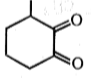(b)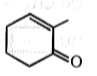(c)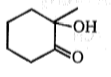(d)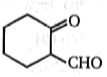High Yielding Test Series + Question Bank - NEET 2020

Difficulty Level:

What is the major product expected from the following reaction?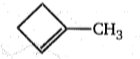$\stackrel{\mathrm{D}-\mathrm{Cl}}{\to }$ Product

1.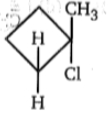2.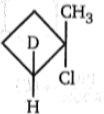3.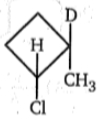4.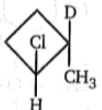High Yielding Test Series + Question Bank - NEET 2020

Difficulty Level: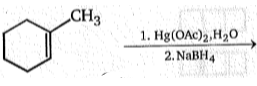Product; Product is:-

(a)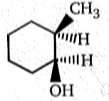(b)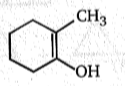(c)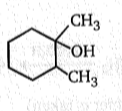(d)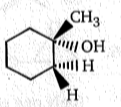High Yielding Test Series + Question Bank - NEET 2020

Difficulty Level:

How many stereoisomeric tetrabromides will be formed in the following reaction  ?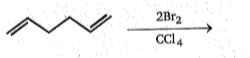(a)2 (b)3 (c) 4 (d) 6

Difficulty Level:

${\mathrm{CH}}_{3}-\underset{|}{\mathrm{C}}\mathrm{H}-{\mathrm{CO}}_{2}\mathrm{K}\phantom{\rule{0ex}{0ex}}{\mathrm{CH}}_{3}-\mathrm{CH}-{\mathrm{CO}}_{2}\mathrm{K}$ $\stackrel{\mathrm{electrolysis}}{\to }$ (A) (Major)

Major product (A) of the above reaction: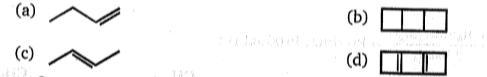High Yielding Test Series + Question Bank - NEET 2020

Difficulty Level: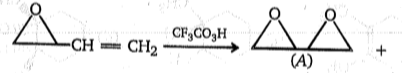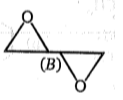(Only one enantiomer is taken)
Which of the following statement is correct about A and B ?
(a) A  and B are mixture of diastereomers
(b) A and B are mixture of enantiomers
(c) A and B are optically active
(d) B is racemic mixture

High Yielding Test Series + Question Bank - NEET 2020

Difficulty Level: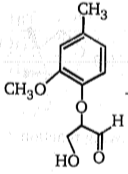$\stackrel{{\mathrm{NaBH}}_{4}}{\to }$A$\stackrel{{\mathrm{O}}_{3}}{\to }$B$\stackrel{{\mathrm{H}}_{2}\mathrm{O}}{\to }$(c) (one of the product)

Identify the product (C):

(a) $\begin{array}{ccccccc}{\mathrm{CH}}_{3}& -& \stackrel{\mathrm{O}}{\stackrel{\parallel }{\mathrm{C}}}& -& \stackrel{\mathrm{O}}{\stackrel{\parallel }{\mathrm{C}}}& -& \mathrm{O}\begin{array}{cc}-& {\mathrm{CH}}_{3}\end{array}\end{array}$

(b) $\begin{array}{ccc}\underset{|}{{\mathrm{CH}}_{2}}& -& \mathrm{OH}\\ \underset{|}{\mathrm{CH}}& -& \mathrm{OH}\\ {\mathrm{CH}}_{2}& -& \mathrm{OH}\end{array}$

(c) $\begin{array}{ccc}\stackrel{\mathrm{CHO}}{\stackrel{|}{\underset{|}{\mathrm{CH}}}}& -& \mathrm{OH}\\ {\mathrm{CH}}_{2}& -& \mathrm{OH}\end{array}$

(d) $\begin{array}{c}\underset{|}{\mathrm{CHO}}\\ \underset{|}{\mathrm{CHOH}}\\ \mathrm{CHO}\end{array}$

High Yielding Test Series + Question Bank - NEET 2020

Difficulty Level: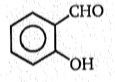$\stackrel{{\mathrm{HO}}^{⊝}}{\to }$ (X) $\stackrel{\mathrm{CH}2=\mathrm{CH}-\stackrel{\oplus }{\mathrm{P}}\mathrm{Ph}3{\mathrm{Br}}^{⊝}}{\to }$ (Y)

Product (Y) of the above reaction is: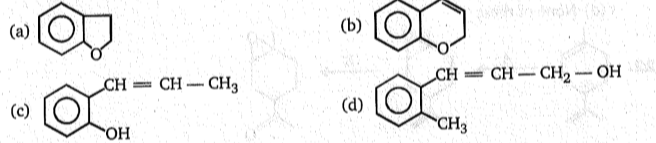High Yielding Test Series + Question Bank - NEET 2020

Difficulty Level: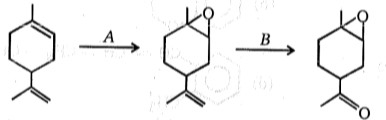Reagent (A) and (B) in the above reaction are:

(a) A = RCO3H, B = H2O2                         (b) A = RCO3H, B = HIO4

(c) A = RCO3H, B = O3                             (d) A = O3, B = RCO3H

High Yielding Test Series + Question Bank - NEET 2020

Difficulty Level:

Rank the following in the increasing order of rate of reaction with HBr.

(P)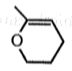(Q)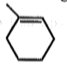(R)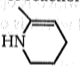(a) R>P>Q

(b) R>Q>P

(c) P>R>S

(d) P>S>R